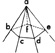Phrases starting with the letter: A B C D E F G H I J K L M N O P Q R S T U V W X Y Z

Previous word: polyhedral
Next word: polyhedron

# Definition of: polyhedral angle

Geom.
The angle formed by three or more planes passing through a point; an angle at a vertex of a solid.POLYHEDRAL ANGLE
Lateral angles bac, bad, etc., form angle at vertex a.

Phrases starting with the letter: A B C D E F G H I J K L M N O P Q R S T U V W X Y Z